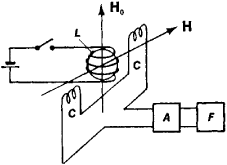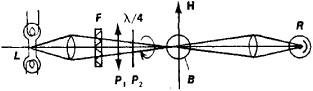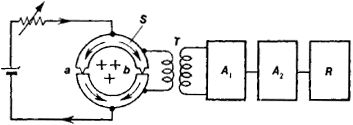Quantum Magnetometer

The following article is from The Great Soviet Encyclopedia (1979). It might be outdated or ideologically biased.

Quantum Magnetometer

an instrument for measuring the intensity of magnetic fields, by utilizing quantum phenomena.

Quantum magnetometers are used primarily to measure the intensity of weak magnetic fields, particularly the earth’s magnetic field and its anomalies, both on the surface and at great altitudes, corresponding to the orbits of ballistic missiles and artificial earth satellites, and also to measure magnetic fields of planets of the solar system and in outer space. They are also used in prospecting for minerals, for magnetic core sampling, and in searching for sunken vessels.

In a magnetic field, the energy levels of particles that have magnetic moments are split into several substates. The energy difference Δε among such substates depends on the intensity H of the magnetic field and in many cases is proportional to it. Particles may pass from one magnetic substate to another, absorbing or emitting a portion (quantum) of electromagnetic energy equal to ℏ γ, where is Planck’s constant and co is the frequency of the electromagnetic field. The frequency co is exactly equal to the frequency of precession of the magnetic moment around the direction of the magnetic field—that is, γ = ϒH, where ϒ is the gyromagnetic ratio. The frequency γ is in the radio-frequency range. The intensity H of the magnetic field can be determined by finding the frequency γ—for example, according to the resonance absorption of radio waves by matter. Since the proportionality constant for the relationship between the frequency co and the field is expressed by atomic constants, which are characterized by extremely high stability and reproducibility, the sensitivity of such quantum magnetometers is very high. The most advanced quantum magnetometers of this type have a sensitivity of up to 10−8 oersted, or 10−3 gamma (1 gamma = 10−5 oersted).

Proton magnetometers. An ampul with a diamagnetic liquid is the sensor in proton magnetometers. The molecules of the liquid (for example, water or benzene) contain hydrogen atoms. The magnetic moments of the molecules are determined solely by the magnetic moments of the nuclei of the hydrogen atoms—that is, by protons (the electron magnetic moments in such liquids are compensated). The ampul is placed in a coil L, through which a current is passed for several seconds, creating an auxiliary magnetic field H0 in the coil; the intensity of this field is several hundred oersteds (Figure 1). As a result of the action of the field H0 the magnetic moments of the protons become oriented and the liquid acquires a total magnetic moment M. After the current is switched off, the magnetic moments of the protons begin to precess around the direction of the magnetic field H being measured, at a frequency γ = γpH, where γp = (2.67513 ± 0.00002) × 104 gauss−1 sec−1. The precession of the total magnetic moment M causes the appearance in the coil C of an alternating electromotive force with a frequency equal to the precession frequency ω. In the earth’s magnetic field, Heℏ 0.6 oersted and ω = 2.55 kilohertz (kHz). Precession is gradually attenuated because of the process of relaxation, which is brought about by the weak interaction between the protons and atoms of paramagnetic additives dissolved in the working fluid. For pure water the relaxation time is about 3 sec. For a second measurement of the field the cycle is repeated. The cyclic nature of the sensor’s operation can be eliminated by the use of a two-sensor system, with alternating operation of the sensors.Figure 1. Diagram of a proton magnetometer: (L) coil generating an auxiliary magnetizing field H0 (C) coil in which an electromotive force arises because of precession of nuclear moments around the magnetic field H being measured, (A) signal amplifier, (F) frequency meter, calibrated in oersteds

Electron magnetometer. The electron quantum magnetometer is analogous to the proton type. It uses the precession in a magnetic field of the unpaired electrons of paramagnetic atoms, the frequency of which is hundreds of times greater than the precession frequency of protons. The precession frequency for electrons in a field H ∼ 1 oersted is 2.8 MHz. A change of 1 gamma in the field causes a change of 28 Hz in the precession frequency, which is 660 times greater than for proton magnetometers.

To produce a sufficiently great electromotive force, methods of dynamic polarization of nuclei are used. The magnetic moments of the protons are oriented as a result of their interaction with the electron moments of paramagnetic ions (a paramagnetic salt dissolved in water). In this way the magnetization of nuclei can be increased by a factor of several hundred. The use of a substance containing the radicals of potassium nitrosodisulfate makes possible an additional increase in magnetization by a factor of about 40.

Optical magnetometer (magnetometer with optical pumping; Figure 2). In an optical magnetometer, a glass bulb is used as the sensor. The bulb is filled with vapors of an alkali metal, such as rubidium, whose atoms are paramagnetic, since they contain one unpaired electron.Figure 2. Diagram of an optical quantum magnetometer: (L) light source, (F) light filter, (P1) polaroid, (P2) plate (λ/4) generating a phase difference of 90° in order to produce circularly polarized light, (B) bulb filled with vapor of an alkali metal, (R) photoreceiver, (H) field being measured

When circularly polarized light whose frequency is equal to the frequency of the optical quantum transition between the ground state of the atom and one of its excited states is passed through the bulb, which is placed in the field H being measured, resonance scattering of light occurs. As this takes place, the angular momentum of the quanta of scattered light is transferred to the atoms, which thus become “optically oriented” and collect on one of the magnetic substates of the ground state. If an alternating magnetic field whose frequency is equal to the frequency of quantum transition between the magnetic substates of the ground state is generated inside the sensor bulb, the atom population of the magnetic substates is equalized, and atoms lose the acquired preferred orientation of magnetic moments and return to their original state. As this takes place, metal vapors filling the bulb again begin strongly to absorb and disperse the light. The intensity of the magnetic field H in which the sensor bulb is located may be determined by measuring the frequency co of the alternating field.

Optical quantum magnetometers are particularly convenient for measurements of weak fields (less than 1 oersted). The sensitivity attainable with instruments of this type is 10−6 to 10−7 oersted, which makes possible the measurement of very weak fields, particularly in outer space.

Superconductor magnetometers. Superconductor magnetometers are based on the quantization of a magnetic flux trapped within a superconducting ring. The magnitude of the trapped flux is a multiple of a quantum of magnetic flux, Φ0 = 2 × 10−7 oersted • cm2. The total current flowing through the parallel connection of two Josephson contacts (a superconducting ring divided along its diameter by a very thin layer of insulation) as a result of the addition of currents flowing through each branch (Figure 3) changes proportional to cos e Φ/ℏ, where Φ is the magnetic flux contained within the ring and e is the charge of an electron. The current attains a maximum whenever Φ = n Φ0 (n is an integer). By observing the changes in the current passing through the double Josephson contact it is possible to measure the magnetic flux Φ and, since the cross-sectional area of the contact is known, to determine the intensity of the field. If the area of two contacts is equal to 1 sq mm, the current maximums are separated by an interval of 2 gammas. An interval one-tenth as large may be recorded by this method. In this case the sensitivity of the method is 0.2 gamma. In the example discussed here the strongest field that could be measured would be about 20 gammas.Figure 3. Diagram of a superconducting magnetometer: (S) superconducting ring with two Josephson contacts (a and b). (1) matching transformer, (A, ) narrow-band amplifier with a detector. (A2) DC amplifier. (R) chart recorder. The magnetic flux through the.ring (perpendicular to the plane of the figure and directed downward) is indicated by the crosses. Changes in the flux cause the appearance of a periodic electromotive force at the input of the amplifier (A1).

Quantum magnetometers are not sensitive to vibration; their readings do not depend on the orientation of the instrument relative to the field H being measured and depend only weakly on changes in temperature, pressure, and humidity.

REFERENCES

Pomerantsev, N. M., V. M. Ryzhkov, and G. V. Skrotskii. Fizicheskie osnovy kvantovoi magnitometrii. Moscow, 1972.
Abragam, A. Iadernyi magnetizm. Moscow, 1963. (Translated from English.)

G. V. SKROTSKII [11–1794–1; updated]